# Registering Incomplete Curves

#### 2022-09-26

This vignette outlines the functionalities of the registr package with regard to incomplete curves.

# 1 Introduction

Incomplete curves arise in many applications. Incompleteness refers to functional data where (some) curves were not observed from the very beginning and/or until the very end of the common domain. Such a data structure is e.g. observed in the presence of drop-out in panel studies.

We differentiate three different types of (in)completeness:

1. no incompleteness,
where processes where all observed from their very beginning until their very end. In this case, it is reasonable to assume that both the starting points and the endpoints of the warping functions in the registration process lie on the diagonal since the observed process is fully comprised in the observed interval.
where processes where not necessarily observed from their very beginning, but until their very end. In this case it is reasonable to assume that the endpoints lie on the diagonal since the observed process is observed until its end. The starting points of the warping functions are able to vary from the diagonal to handle potential time distortions towards the beginning of the observed domains.
3. trailing incompleteness,
where processes where observed from their very beginning, but not necessarily until their very end. In this case it is reasonable to assume that the starting points lie on the diagonal since the observed process is observed from its beginning. The endpoints of the warping functions are able to vary from the diagonal to handle potential time distortions towards the end of the observed domains.
4. full incompleteness,
where processes where neither necessarily observed from their very beginning, nor until their very end. In this case it is not reasonable to assume that either the starting points or the endpoints lie on the diagonal. The starting points and the endpoints of the warping functions are able to vary from the diagonal to handle potential time distortions both towards the beginning and the end of the observed domains.

Exemplarily, we showcase the following functionalities on data from the Berkeley Growth Study (see ?growth_incomplete) where we artificially simulated that not all children were observed right from the start and that a relevant part of the children dropped out early of the study at some point in time:

dat = registr::growth_incomplete

# sort the data by the amount of trailing incompleteness
ids    = levels(dat$id) dat$id = factor(dat$id, levels = ids[order(sapply(ids, function(curve_id) { max(dat$index[datid == curve_id]) }))]) if (have_ggplot2) { # spaghetti plot ggplot(dat, aes(x = index, y = value, group = id)) + geom_line(alpha = 0.2) + xlab("t* [observed]") + ylab("Derivative") + ggtitle("First derivative of growth curves") }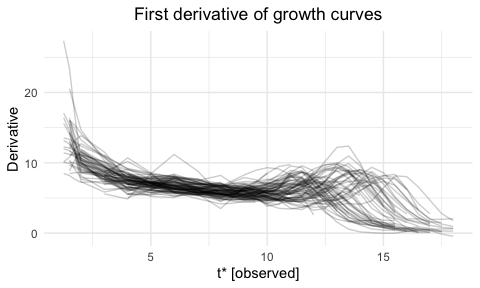if (have_ggplot2) { ggplot(dat, aes(x = index, y = id, col = value)) + geom_line(lwd = 2.5) + scale_color_continuous("Derivative", high = "midnightblue", low = "lightskyblue1") + xlab("t* [observed]") + ylab("curve") + ggtitle("First derivative of growth curves") + theme(panel.grid = element_blank(), axis.text.y = element_blank()) }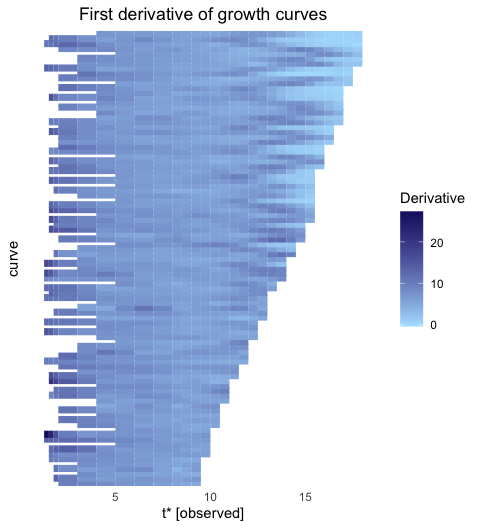# 2 Incomplete curve methodology We adapt the registration methodology outlined in Wrobel et al. (2019) to handle incomplete curves. Since each curve potentially has an individual range of its observed time domain, the spline basis for estimating a curve’s warping function is defined individually for each curve, based on a given number of basis functions. It often is a quite strict assumption in incomplete data settings that all warping functions start and/or end on the diagonal, i.e. that the individual, observed part of the whole time domain is not (to some extent) distorted. Therefore, the registr package gives the additional option to estimate warping functions without the constraint that their starting point and/or endpoint lies on the diagonal. On the other hand, if we fully remove such constraints, this can result in very extreme and unrealistic distortions of the time domain. This problem is further accompanied by the fact that the assessment of some given warping to be realistic or unrealistic can heavily vary between different applications. As of this reason, our method includes a penalization parameter $$\lambda$$ that has to be set manually to specify which kinds of distortions are deemed realistic in the application at hand. Mathematically speaking, we add a penalization term to the likelihood $$\ell(i)$$ for curve $$i$$. For a setting with full incompleteness (i.e., where both the starting point and endpoint are free to vary from the diagonal) this results in \begin{aligned} \ell_{\text{pen}}(i) &= \ell(i) - \lambda \cdot n_i \cdot \text{pen}(i), \\ \text{with} \ \ \ \text{pen}(i) &= \left( \left[\hat{h}_i^{-1}(t_{max,i}^*) - \hat{h}_i^{-1}(t_{min,i}^*)\right] - \left[t_{max,i}^* - t_{min,i}^*\right] \right)^2, \end{aligned} where $$t^*_{min,i},t^*_{max,i}$$ are the minimum / maximum of the observed time domain of curve $$i$$ and $$\hat{h}^{-1}_i(t^*_{min,i}), \hat{h}^{-1}_i(t^*_{max,i})$$ the inverse warping function evaluated at this minimum / maximum. For leading incompleteness with $$h_i^{-1}(t_{max,i}^*) = t_{max,i}^* \forall i$$ this simplifies to $$\text{pen}(i) = \left(\hat{h}_i^{-1}(t_{min,i}^*) - t_{min,i}^*\right)^2$$, and for trailing incompleteness with $$h_i^{-1}(t_{min,i}^*) = t_{min,i}^* \forall i$$ to $$\text{pen}(i) = \left(\hat{h}_i^{-1}(t_{max,i}^*) - t_{max,i}^*\right)^2$$. The penalization term is scaled by the number of measurements $$n_i$$ of curve $$i$$ to ensure a similar impact of the penalization for curves with different numbers of measurements. The higher the penalization parameter $$\lambda$$, the more the length of the registered domain is forced towards the length of the observed domain. Given a specific application, $$\lambda$$ should be chosen s.t. unrealistic distortions of the time domain are prevented. To do so, the user has to run the registration approach multiple times with different $$\lambda$$’s to find an optimal value. # 3 Application on incomplete growth data By default, both functions register_fpca and registr include the argument incompleteness = NULL to constrain all warping functions to start and end on the diagonal. reg1 = registr(Y = dat, family = "gaussian") if (have_ggplot2) { ggplot(reg1Y, aes(x = tstar, y = index, group = id)) +
geom_line(alpha = 0.2) +
xlab("t* [observed]") + ylab("t [registered]") +
ggtitle("Estimated warping functions")
}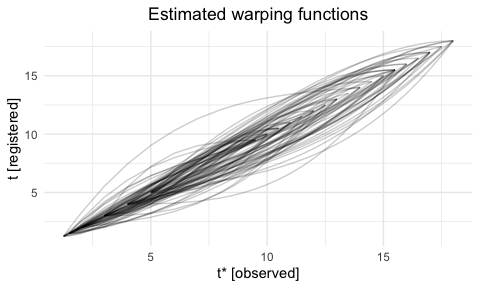if (have_ggplot2) {
ggplot(reg1$Y, aes(x = index, y = id, col = value)) + geom_line(lwd = 2.5) + scale_color_continuous("Derivative", high = "midnightblue", low = "lightskyblue1") + xlab("t [registered]") + ylab("curve") + ggtitle("Registered curves") + theme(panel.grid = element_blank(), axis.text.y = element_blank()) }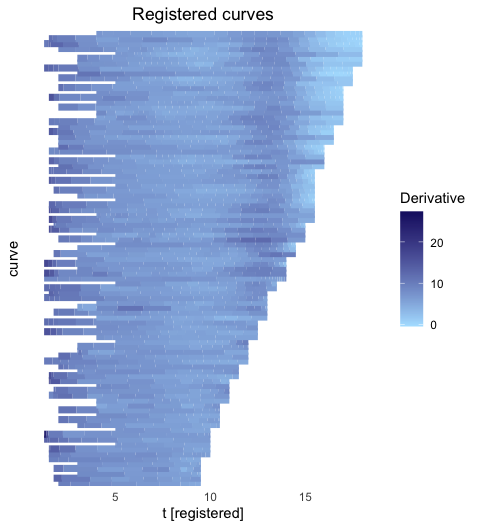if (have_ggplot2) { ggplot(reg1$Y, aes(x = index, y = value, group = id)) +
geom_line(alpha = 0.3) +
xlab("t [registered]") + ylab("Derivative") +
ggtitle("Registered curves")
}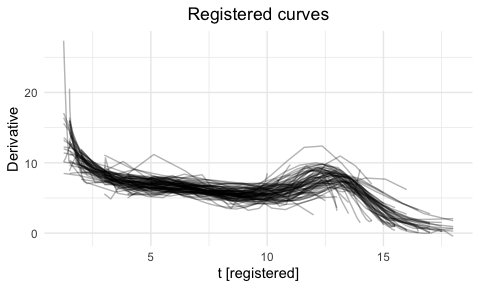The assumption can be dropped by setting incompleteness to some other value than NULL and some nonnegative value for the penalization parameter lambda_inc. The higher lambda_inc is chosen, the more the registered domains are forced to have the same length as the observed domains.

### 3.0.1 Small lambda_inc

reg2 = registr(Y = dat, family = "gaussian",
incompleteness = "full", lambda_inc = 0)

if (have_ggplot2) {
ggplot(reg2$Y, aes(x = tstar, y = index, group = id)) + geom_line(alpha = 0.2) + xlab("t* [observed]") + ylab("t [registered]") + ggtitle("Estimated warping functions") }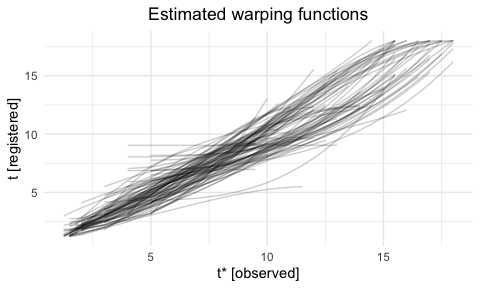if (have_ggplot2) { ggplot(reg2$Y, aes(x = index, y = id, col = value)) +
geom_line(lwd = 2.5) +
scale_color_continuous("Derivative", high = "midnightblue", low = "lightskyblue1") +
xlab("t [registered]") + ylab("curve") +
ggtitle("Registered curves") +
theme(panel.grid  = element_blank(),
axis.text.y = element_blank())
}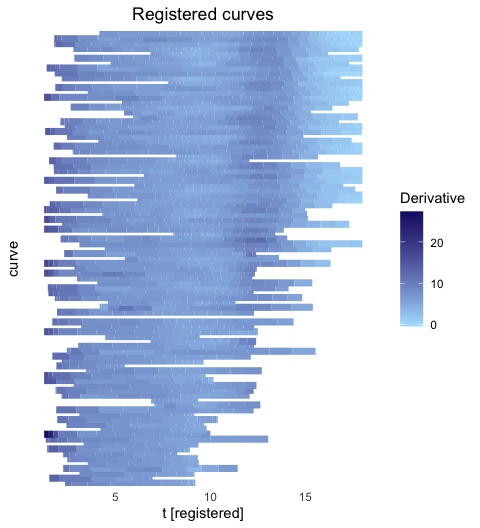if (have_ggplot2) {
ggplot(reg2$Y, aes(x = index, y = value, group = id)) + geom_line(alpha = 0.3) + xlab("t [registered]") + ylab("Derivative") + ggtitle("Registered curves") }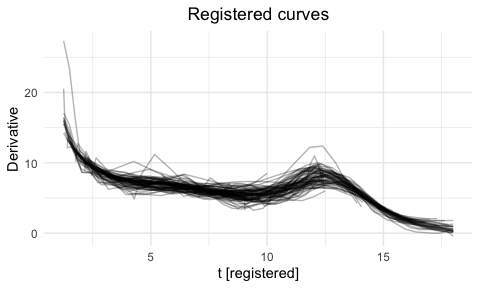### 3.0.2 Larger lambda_inc reg3 = registr(Y = dat, family = "gaussian", incompleteness = "full", lambda_inc = 5) if (have_ggplot2) { ggplot(reg3$Y, aes(x = tstar, y = index, group = id)) +
geom_line(alpha = 0.2) +
xlab("t* [observed]") + ylab("t [registered]") +
ggtitle("Estimated warping functions")
}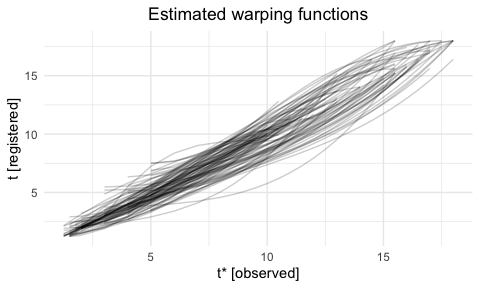if (have_ggplot2) {
ggplot(reg3$Y, aes(x = index, y = id, col = value)) + geom_line(lwd = 2.5) + scale_color_continuous("Derivative", high = "midnightblue", low = "lightskyblue1") + xlab("t [registered]") + ylab("curve") + ggtitle("Registered curves") + theme(panel.grid = element_blank(), axis.text.y = element_blank()) }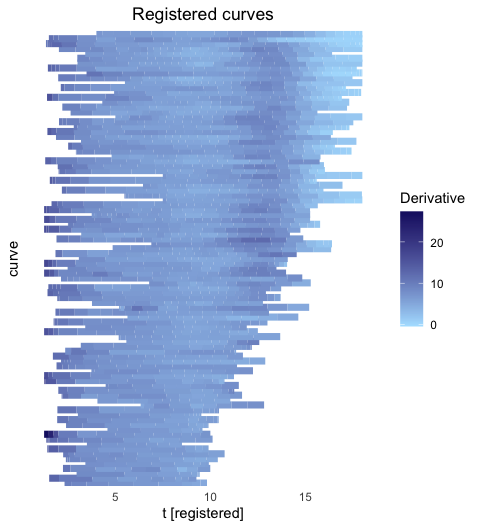if (have_ggplot2) { ggplot(reg3$Y, aes(x = index, y = value, group = id)) +
geom_line(alpha = 0.3) +
xlab("t [registered]") + ylab("Derivative") +
ggtitle("Registered curves")
}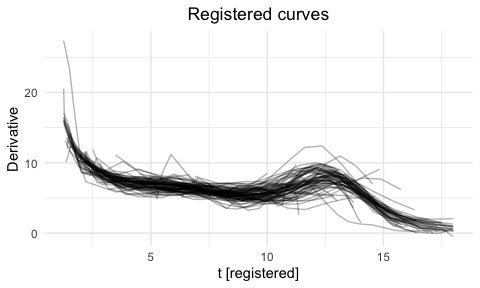### 3.0.3 Choosing an optimal lambda_inc

As outlined, $$\lambda$$ should be set to the smallest value that prevents unrealistic distortions of the time domain. The intuition of what kinds of distortions are unrealistic has to be based on subject knowledge.

In the above example we see that lambda_inc = 0 leads to extreme compressions of the time domain, which can clearly be viewed as unrealistic. These compressions can for example be prevented by setting lambda_inc = 0.025.

reg4 = registr(Y = dat, family = "gaussian",
incompleteness = "full", lambda_inc = .025)

if (have_ggplot2) {
ggplot(reg4$Y, aes(x = tstar, y = index, group = id)) + geom_line(alpha = 0.2) + xlab("t* [observed]") + ylab("t [registered]") + ggtitle("Estimated warping functions") }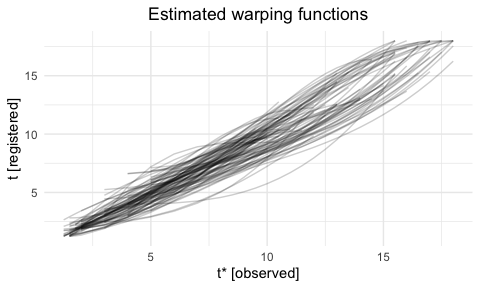Underlying functional principal components can be estimated jointly to the registration by calling register_fpca() and visualizing them with registr:::plot.fpca(). reg4_joint = register_fpca(Y = dat, family = "gaussian", incompleteness = "full", lambda_inc = .025, npc = 4) if (have_ggplot2) { ggplot(reg4_joint$Y, aes(x = t_hat, y = value, group = id)) +
geom_line(alpha = 0.3) +
xlab("t [registered]") + ylab("Derivative") +
ggtitle("Registered curves")
}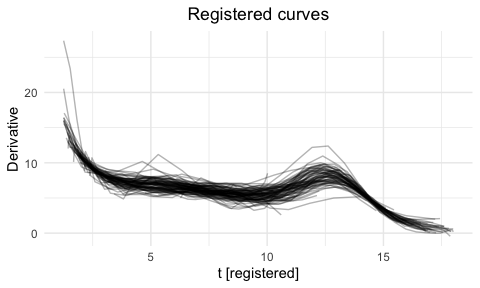if (have_ggplot2) {
plot(reg4_joint\$fpca_obj)
}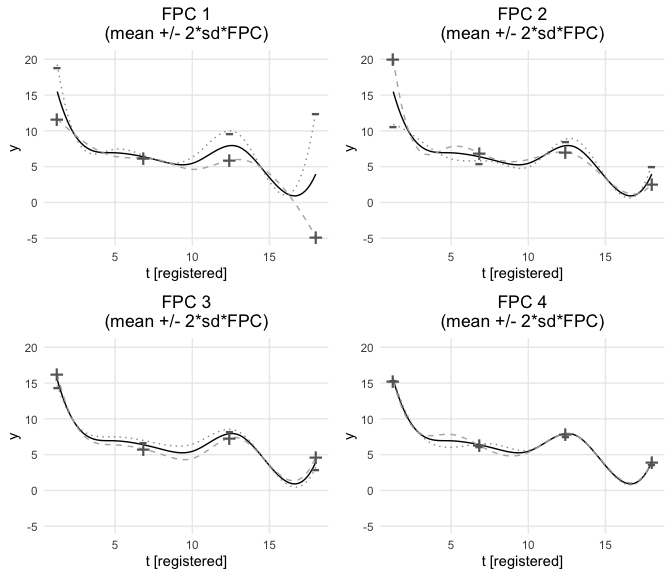# 4 Constraint matrices for the optimization

Warping functions are estimated using the function constrOptim(). For the estimation of the warping function for curve $$i$$ it uses linear inequality constraints of the form $\boldsymbol{u}_i \cdot \boldsymbol{\beta}_i - \boldsymbol{c}_i \geq \boldsymbol{0},$ where $$\boldsymbol{\beta}_i$$ is the parameter vector and matrix $$\boldsymbol{u}_i$$ and vector $$\boldsymbol{c}_i$$ define the constraints.

For the estimation of a warping function the parameter vector is constrained s.t. the resulting warping function is monotone and does not exceed the overall time domain $$[t_{min},t_{max}]$$.

In the following the constraint matrices are listed for the different settings of (in)completeness and assuming a parameter vector of length $$p$$: $\boldsymbol{\beta}_i = \left( \begin{array}{c} \beta_{i1} \\ \beta_{i2} \\ \vdots \\ \beta_{ip} \end{array} \right) \in \mathbb{R}_{p \times 1}$

Note:
All following constraint matrices refer to the estimation of nonparametric inverse warping functions with warping = "nonparametric".

## 4.1 Complete curve setting

When all curves were observed completely – i.e. the underlying processes of interest were all observed from the beginning until the end – warping functions can typically be assumed to start and end on the diagonal, since each process is completely observed in its observation interval $$[t^*_{min,i},t^*_{max,i}] \subset [t_{min},t_{max}]$$.

Assuming that both the starting point and the endpoint lie on the diagonal, we set $$\beta_{i1} = t^*_{min,i}$$ and $$\beta_{ip} = t^*_{max,i}$$ and only perform the estimation for $\left( \begin{array}{c} \beta_{i2} \\ \beta_{i3} \\ \vdots \\ \beta_{i(p-1)} \end{array} \right) \in \mathbb{R}_{(p-2) \times 1}$

This results in the following constraint matrices, that allow a mapping from the observed domain $$[t^*_{min,i},t^*_{max,i}]$$ to the domain itself $$[t^*_{min,i},t^*_{max,i}] \subset [t_{min},t_{max}]$$: \begin{aligned} \boldsymbol{u}_i &= \left( \begin{array}{cccccccc} 1 & 0 & 0 & 0 & \ldots & 0 & 0 & 0 \\ -1 & 1 & 0 & 0 & \ldots & 0 & 0 & 0 \\ 0 & -1 & 1 & 0 & \ldots & 0 & 0 & 0 \\ \vdots & \ddots & \ddots & \ddots & \ddots & \ddots & \ddots & \ddots \\ 0 & 0 & 0 & 0 & \ldots & 0 & -1 & 1 \\ 0 & 0 & 0 & 0 & \ldots & 0 & 0 & -1 \end{array} \right) \in \mathbb{R}_{(p-1) \times (p-2)} \\ \boldsymbol{c}_i &= \left( \begin{array}{c} t^*_{min,i} \\ 0 \\ 0 \\ \vdots \\ 0 \\ -1 \cdot t^*_{max,i} \end{array} \right) \in \mathbb{R}_{(p-1) \times 1} \end{aligned}

In the case of leading incompleteness – i.e. the underlying processes of interest were all observed until their very end but not necessarily starting from their beginning – warping functions can typically be assumed to end on the diagonal, s.t. one assumes $$\beta_{ip} = t^*_{max,i}$$ to let the warping functions end at the last observed time point $$t^*_{max,i}$$. The estimation is then performed for the remaining parameter vector $\left( \begin{array}{c} \beta_{i1} \\ \beta_{i3} \\ \vdots \\ \beta_{i(p-1)} \end{array} \right) \in \mathbb{R}_{(p-1) \times 1}$

This results in the following constraint matrices, that allow a mapping from the observed domain $$[t^*_{min,i},t^*_{max,i}]$$ to the domain $$[t_{min},t^*_{max,i}] \subset [t_{min},t_{max}]$$: \begin{aligned} \boldsymbol{u}_i &= \left( \begin{array}{cccccccc} 1 & 0 & 0 & 0 & \ldots & 0 & 0 & 0 \\ -1 & 1 & 0 & 0 & \ldots & 0 & 0 & 0 \\ 0 & -1 & 1 & 0 & \ldots & 0 & 0 & 0 \\ \vdots & \ddots & \ddots & \ddots & \ddots & \ddots & \ddots & \ddots \\ 0 & 0 & 0 & 0 & \ldots & 0 & -1 & 1 \\ 0 & 0 & 0 & 0 & \ldots & 0 & 0 & -1 \end{array} \right) \in \mathbb{R}_{p \times (p-1)} \\ \boldsymbol{c}_i &= \left( \begin{array}{c} t_{min} \\ 0 \\ 0 \\ \vdots \\ 0 \\ -1 \cdot t^*_{max,i} \end{array} \right) \in \mathbb{R}_{p \times 1} \end{aligned}

## 4.3 Trailing incompleteness only

In the case of trailing incompleteness – i.e. the underlying processes of interest were all observed from the beginning but not necessarily until their very end – warping functions can typically be assumed to start on the diagonal, s.t. one assumes $$\beta_{i1} = t^*_{min,i}$$ to let the warping functions start at the first observed time point $$t^*_{min,i}$$. The estimation is then performed for the remaining parameter vector $\left( \begin{array}{c} \beta_{i2} \\ \beta_{i3} \\ \vdots \\ \beta_{ip} \end{array} \right) \in \mathbb{R}_{(p-1) \times 1}$

This results in the following constraint matrices, that allow a mapping from the observed domain $$[t^*_{min,i},t^*_{max,i}]$$ to the domain $$[t^*_{min,i},t_{max}] \subset [t_{min},t_{max}]$$: \begin{aligned} \boldsymbol{u}_i &\text{ identical to the version for leading incompleteness} \\ \boldsymbol{c}_i &= \left( \begin{array}{c} t^*_{min,i} \\ 0 \\ 0 \\ \vdots \\ 0 \\ -1 \cdot t_{max} \end{array} \right) \in \mathbb{R}_{p \times 1} \end{aligned}

## 4.4 Leading and trailing incompleteness

In the case of both leading and trailing incompleteness – i.e. the underlying processes of interest were neither necessarily observed from their very beginnings nor to their very ends – warping functions can typically only be assumed to map the observed domains $$[t^*_{min,i},t^*_{max,i}]$$ to the overall domain $$[t_{min},t_{max}]$$.

This results in the following constraint matrices: \begin{aligned} \boldsymbol{u}_i &= \left( \begin{array}{cccccccc} 1 & 0 & 0 & 0 & \ldots & 0 & 0 & 0 \\ -1 & 1 & 0 & 0 & \ldots & 0 & 0 & 0 \\ 0 & -1 & 1 & 0 & \ldots & 0 & 0 & 0 \\ \vdots & \ddots & \ddots & \ddots & \ddots & \ddots & \ddots & \ddots \\ 0 & 0 & 0 & 0 & \ldots & 0 & -1 & 1 \\ 0 & 0 & 0 & 0 & \ldots & 0 & 0 & -1 \end{array} \right) \in \mathbb{R}_{(p+1) \times p} \\ \boldsymbol{c}_i &= \left( \begin{array}{c} t_{min} \\ 0 \\ 0 \\ \vdots \\ 0 \\ -1 \cdot t_{max} \end{array} \right) \in \mathbb{R}_{(p+1) \times 1} \end{aligned}

# 5 Help files

Documentation for individual functions gives more information on their arguments and return objects, and can be pulled up via the following:

• ?register_fpca
• ?registr